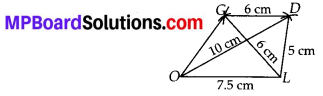# MP Board Class 8th Maths Solutions Chapter 4 Practical Geometry Ex 4.2

## MP Board Class 8th Maths Solutions Chapter 4 Practical Geometry Ex 4.2

Question 1.
LIFT
LI = 4 cm
IF = 3 cm
TL = 2.5 cm
LF= 4.5 cm
IT =4 cm

GOLD
OL = 7.5 cm
GL = 6 cm
GD = 6 cm
LD = 5 cm
OD = 10 cm(iii) Rhombus BEND
BN = 5.6 cm,
DE = 6.5 cm
Solution:
(i) Steps of Construction:
Step-1: Draw LI = 4 cm.
Step-2: With L as centre and radius equal to 2.5 cm, cut an arc.
Step-3: With I as centre and radius equal to 4 cm, cut another arc on the arc drawn in step-2 at point T.
Step-4 : With I as centre and radius equal to 3 cm, cut an arc.
Step-5: With L as centre and radius equal to 4.5 cm, cut another arc on the arc drawn in step-4 at point F.
Step-6 : Join LT, IT, LF, IF and TF.
Hence, LIFT is the required quadrilateral.(ii) Steps of Construction:
Step-1: Draw OL = 7.5 cm.
Step-2: With L as centre and radius equal to 5 cm cut an arc.
Step-3 : With O as centre and radius equal to 10 cm, cut another arc on the arc drawn in step-2 at point D.
Step-4: With L as centre and radius equal to 6 cm, cut another arc.
Step-5: With D as centre and radius equal to 6 cm cut an arc on arc drawn in step-4 at point G.
Step-6 : Join LD, LG, OG, OD and DG. Hence, GOLD is the required quadrilateral.(iii) Here, BN = 5.6 cm and DE = 6.5 cm are given. These two sides are diagonals of a rhombus BEND. We know that diagonals of a rhombus bisect each other at right angles.

Steps of Construction:
Step-1: Draw BN = 5.6 cm.
Step-2 : Draw perpendicular bisector XY of BN which intersect BN at O.
Step-3 : Cut off OD = OF = 3.25 cm on $$\overrightarrow{\mathrm{OX}}$$ and $$\overrightarrow{\mathrm{OY}}$$ respectively.
Step-4 : Join BD, ND, BE and NE.
Hence, BEND is the required rhombus.